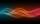# 15 number

What number is smaller (greater) by 15 than its half?

Result

a =  -30
b =  30

#### Solution:

a+15=a/2
b-15=b/2

a = -30
b = 30

a = -30
b = 30

Calculated by our linear equations calculator.

Leave us a comment of this math problem and its solution (i.e. if it is still somewhat unclear...):Be the first to comment!#### Following knowledge from mathematics are needed to solve this word math problem:

Do you have a linear equation or system of equations and looking for its solution? Or do you have quadratic equation?

## Next similar math problems:

1. Dropped sheetsThree consecutive sheets dropped from the book. The sum of the numbers on the pages of the dropped sheets is 273. What number has the last page of the dropped sheets?
2. Unknown number 11That number increased by three equals three times itself?
3. Mysterious numberThe magician thinks the number: "The mysterious number is first divided by minus five, dividing the result by three, multiplying the number by ten, and dividing the resulting number by minus four. This gives result 5. Can you reveal the mysterious number?
4. Unknown numberI think the number. I'll reduce it to its one-third. The result is then increased by one-third, and I get the number 12.
5. Equation 11Solve equation: ?
6. Negative in equation2x + 3 + 7x = – 24, what is the value of x?
7. Simple equation 1035= 7*3*x what is x?
8. NormThree workers planted 3555 seedlings of tomatoes in one dey. First worked at the standard norm, the second planted 120 seedlings more and the third 135 seedlings more than the first worker. How many seedlings were standard norm?
9. Simple equationSolve the following simple equation: 2. (4x + 3) = 2-5. (1-x)
10. Simple equation 8Solve the following equation: 36=-(1+7x)-6(-7-x)
11. Forest nurseryIn the forest nursery after winter, they found that 1/10 stems died out of them. For them, they land 193 new spruces. How many spruces are in the forest nursery?
12. Simple equation 9Solve the following equation: -8y+5=-9y+9
13. Find xSolve: if 2(x-1)=14, then x= (solve an equation with one unknown)
14. Equation 29Solve next equation: 2 ( 2x + 3 ) = 8 ( 1 - x) -5 ( x -2 )
15. EquatiomSolve equation with negatives: X/(-5) + 2 = -9
16. Simple equationSolve for x: 3(x + 2) = x - 18
17. EquationFind x: x + 1/2 = 1/3